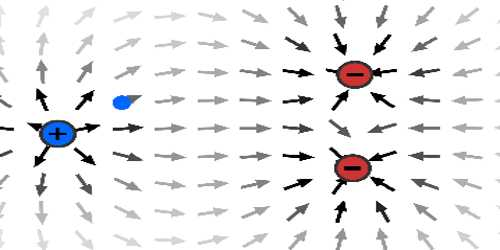# Electric Field

Before discussing electric field let define a test charge., “An imaginary charge of exceedingly small magnitude whose presence does not affect other charges i.e. does not influence the neighboring charges is called a test charge.”

Electric field: An electric field is a force field that surrounds electric charges that attracts or repels other electric charges. Influence of a point charge is experienced around a large space around it [Figure]. If a test charge is placed in that space, it experiences an electric force. This force becomes larger as the test charge approaches towards the point charge and gets weaker as the distance increases and becomes so negligible at long distance that it cannot be measured. From Coulomb’s law, we know that the electric force, like gravitational force, varies inversely to the square of the distance and both the forces are extended to an infinite distance, although if the distance is extended very much the magnitude of force becomes very little and it is not possible to measure it.As mentioned earlier that when a test charge is brought near a point charge, it experiences a force. Now a question arises why and how this force acts when there is no physical contact between the two charges. Famous scientist Michael Faraday first realized that around a charge some sort of disturbance is created in the surrounding space. As a result, if a test charge is placed in that space it experiences the force. He called this disturbance electric field. So electric held can be defined in the following way.

The space around a charged body where its influence is experienced is called the electric field of that charged body.

Unit of the electric field: In S. I. system, the unit of force is Newton and unit of charge is coulomb. So, the unit of an electric field will be Newton/Coulomb (N/C). Besides, there is another unit. That is volt/meter (V/m). Considering the gradient of the electric field it can be written as E = dV/dx. So volt/meter unit is extensively used.